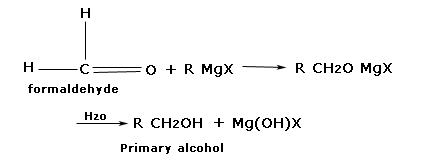## General method of preparation of Alcohol

Subject: Chemistry

#### Overview

Haloalkane reacts with aueous alkali or moist AgO to give corresponding alcohols. $$\underbrace{R-H}_{halo\,Alkane}\xrightarrow{aq.alkali\,or\,moist\,Ag_2O}\underbrace{R-OH}_{Alcohol}$$ Aldehydes and ketones from addition product with Grignard reagent. These addition products of hydrolysis in presence of an acid give alcohols. All three types of alcohol )10, 20, and 30) can be prepared by this method.Primary amines react with nitrous acid to give corresponding alcohol. $$\underbrace{R-NH_2}_{Primary\,amine}+HO-NO→\underbrace{R-OH}_{Alcohol}+N_2+H_2O$$

### 1. From haloalkane.

Haloalkane reacts with aueous alkali or moist AgO to give corresponding alcohols.

$$\underbrace{R-H}_{halo\,Alkane}\xrightarrow{aq.alkali\,or\,moist\,Ag_2O}\underbrace{R-OH}_{Alcohol}$$

Example.

$$\underbrace{CH_3CH_2Cl}_{Chloro\,ethane}\xrightarrow{aq.KOH}\underbrace{CH_3CH_2OH}_{Ethanol}+KCl$$

$$\underbrace{CH_3-CH-BR-CH_3}_{2-bromo\,propane}+Ag_2O(Moist)→\underbrace{CH_3-CH-OH-CH_3}_{2-propanol}+2AgBr$$

#### 2. From carbonyl compounds.

Carbonyl compounds C=O like aldehyde and ketones as well as other organic compounds such as carboxylic acid, esters, anhydrides are reduced to alcohols by the action of LiAlH4, NaBH4, or H2/Ni

$$\underbrace{C=O}_{Carbonyl\,Compound}+2[H]\xrightarrow{reducing\,agent}\underbrace{CH-OH}_{Alcohol}$$

Example.

$$\underbrace{CH_3-COH}_{Ethanal\,or\,Acetaldehyde)}+2[H]\xrightarrow{LiAlH_4}\underbrace{CH_3CH_2OH}_{Ethanol}$$$$\underbrace{CH_3-CO-CH_3}_{Propanone\,or\,Acetone}+2[H]\xrightarrow{H_2\,Ni}\underbrace{CH_3-CH-OH-CH_3}_{2-Propanol}$$

$$\underbrace{CH_3-COOH}_{Ethanoic\,acid\,or\,Acetic\,acid}+H_2\xrightarrow{Ni}\underbrace{CH_3-CH_2-OH}_{Ethanol}+H_2O$$

$$\underbrace{CH_3-COOC_2H_5}_{Ethyl\,ethanoate\,or\,Ethyl\,acetate}\xrightarrow{LiAlH_4}\underbrace{2CH_3-CH_2OH}_{Ethanol}$$

3. From Grignards reagent.

Aldehydes and ketones from addition product with Grignard reagent. These addition products of hydrolysis in presence of an acid give alcohols. All three types of alcohol )10, 20, and 30) can be prepared by this method.

Preparation of primary alcohol.

Formaldehyde produces addition products with Grignard reagent which on hydrolysis from alcoholExample2 preparation of secondary alcohol.

All other aldehydes except formaldehyde produce secondary alcohol when treated with Grignard reagent followed by hydrolysis.Example.

3. Preparation of Tertiary alcohol.

Example.

4. From primary amines.

Primary amines react with nitrous acid to give corresponding alcohol.

$$\underbrace{R-NH_2}_{Primary\,amine}+HO-NO→\underbrace{R-OH}_{Alcohol}+N_2+H_2O$$

Example.

$$\underbrace{CH_3CH_2NH_2}_{Ethanamine}+HO-NO→\underbrace{CH_3-CH_2-OH}_{Ethanol}+N_2+H_2O$$

5. By hydrolysis of ester.

Esters can be hydrolyzed both in acidic and basic medium to give alcohol and carboxylic acid.

$$\underbrace{R-C=O-OR'}_{Ester}+H-OH\xrightarrow{H^+\,or\,OH^-}\underbrace{R-C=O-OH}_{Carboxylic\,acid}+\underbrace{R'-OH}_{Alcohol}$$

Reference.

Bahl, B S, Bahl, and Arun. Advanced Organic chemistry. S. Chand and company Ltd., n.d.

Sthapit, M K, R R Pradhananga, and K B Bajracharya. Foundations of chemistry. Taleju Prakashan, n.d.

Tewari, K S, S N Mehrotra, and N K Vishnoi. A textbook of organic chemistry. Vikash publishing House Pvt. ltd., n.d.

Verma, N K and S K Khanna. Compressive chemistry. 8th edition. Laxmi publications P. Ltd., 1999.

##### Things to remember
1. Haloalkane reacts with aqueous alkali or moist AgO to give corresponding alcohols.

$$\underbrace{R-H}_{halo\,Alkane}\xrightarrow{aq.alkali\,or\,moist\,Ag_2O}\underbrace{R-OH}_{Alcohol}$$

2. Carbonyl compounds C=O like aldehyde and ketones as well as other organic compounds such as carboxylic acid, esters, anhydrides are reduced to alcohols by the action of LiAlH4, NaBH4, or H2/Ni

3. $$\underbrace{C=O}_{Carbonyl\,Compound}+2[H]\xrightarrow{reducing\,agent}\underbrace{CH-OH}_{Alcohol}$$

• It includes every relationship which established among the people.
• There can be more than one community in a society. Community smaller than society.
• It is a network of social relationships which cannot see or touched.
• common interests and common objectives are not necessary for society.